Maths-
General
Easy

Question

# The equation 2x + 7 represents a north path on a map. Find the equation for a path that passes through the point (6, 3) and is perpendicular to the north path.Hint:

## The correct answer is: y = -1/2x + 6

### We have been given an equation that represents the north path on a map. We have to find an equation for a path that passes through the point (6, 3) and is perpendicular to the north path. Step 1 of 1:We have given a line passes through a point (6, 3) and perpendicular to a line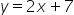Since product of two perpendicular lines is equal to -1.So,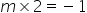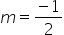Therefore the equation of the line will be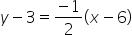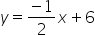#### With Turito Foundation.#### Get an Expert Advice From Turito.